• 二、任意角的三角函数 二(1)、三角函数的定义 二(2)、三角函数线 二(3)、同角三角函数的基本关系 二(4)、诱导公式 三、三角函数的图像与性质 二(1)正弦函数、余弦函数的图像与性质 二(1)1 正弦函数、余弦函数的图像...

查看目录
一、任意角的概念与弧度制
一(1)、任意角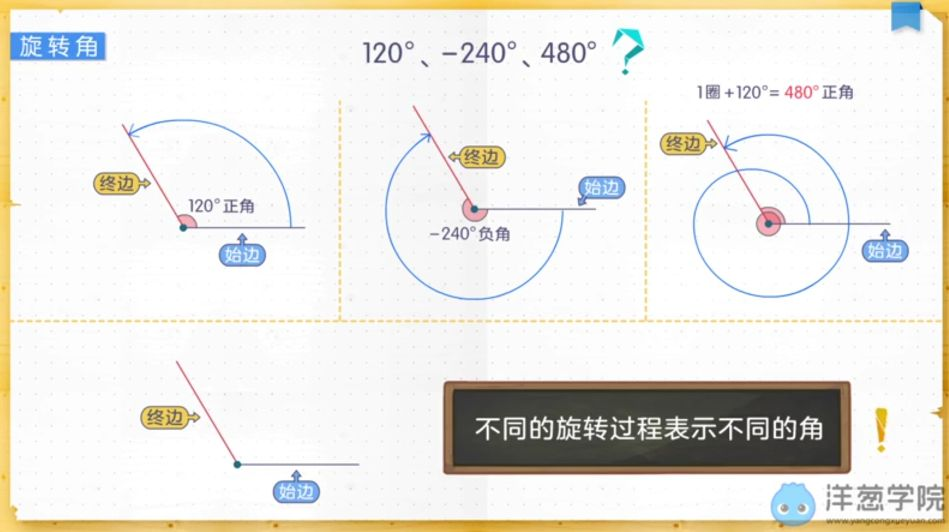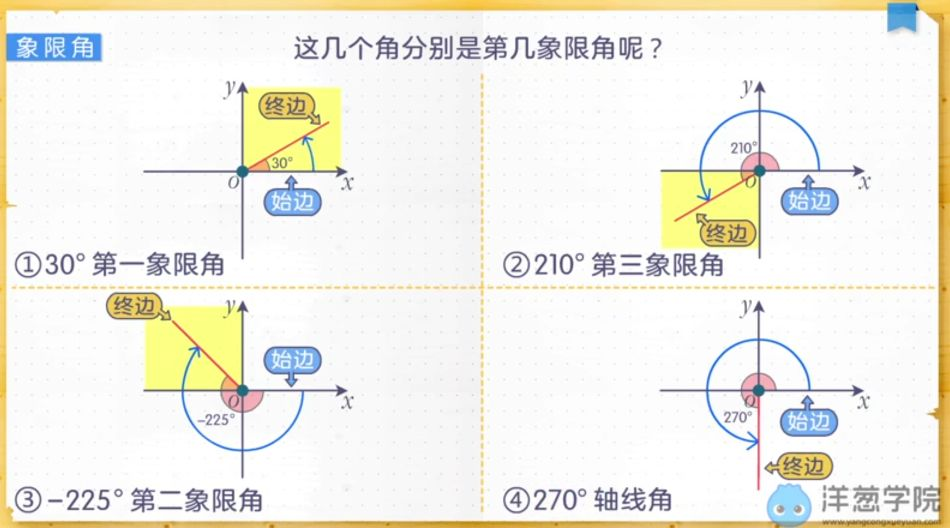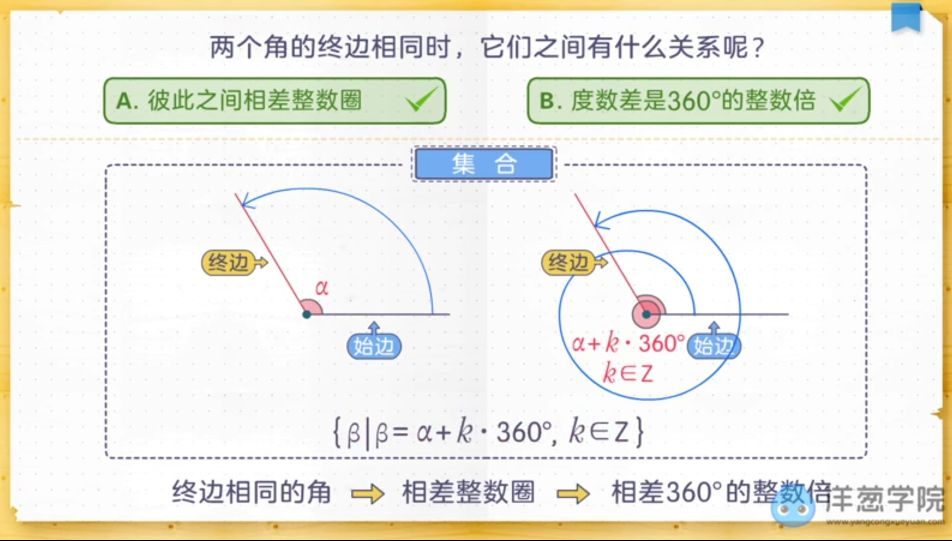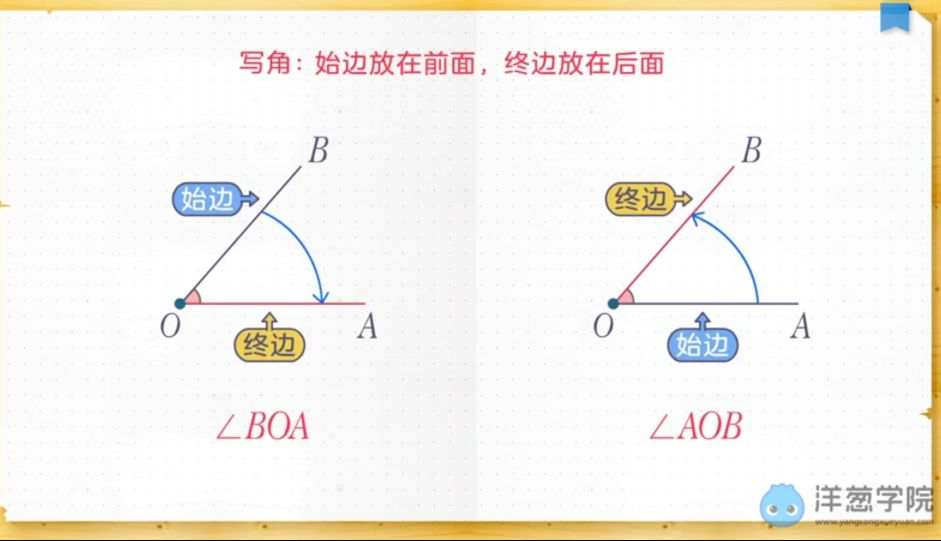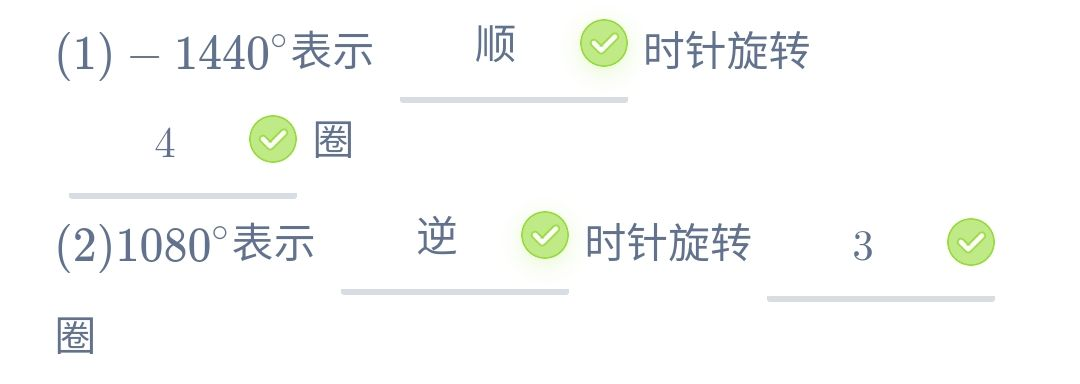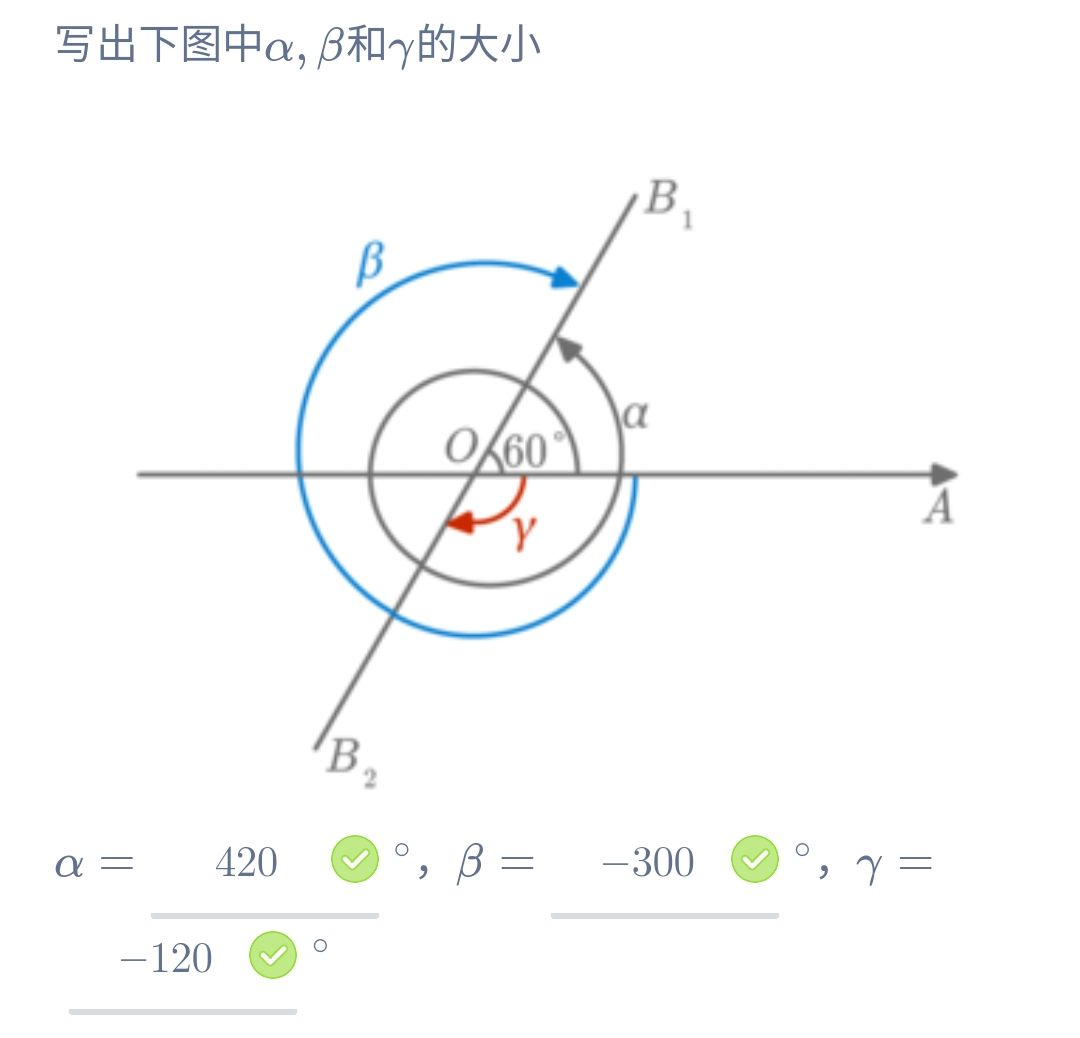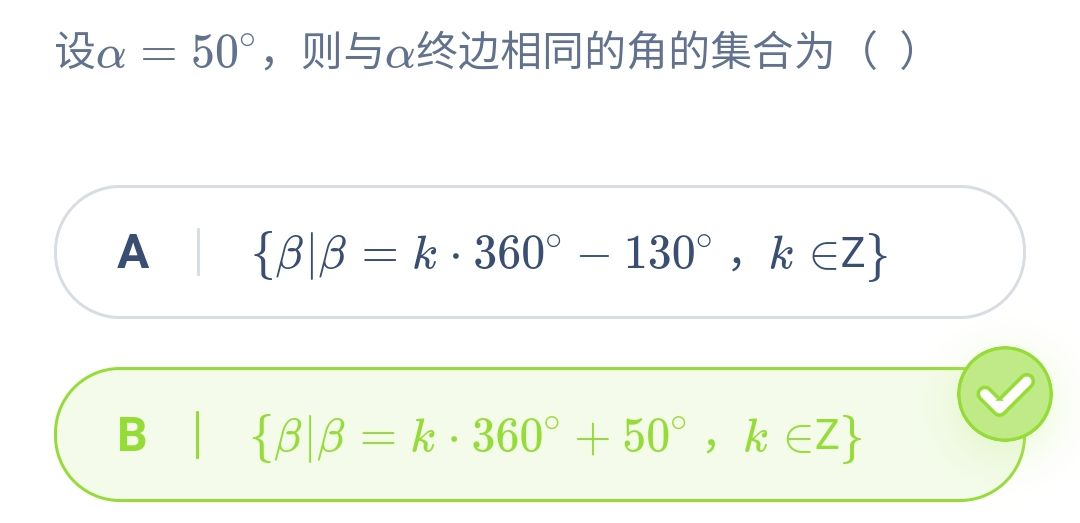一(2)、角度制与弧度制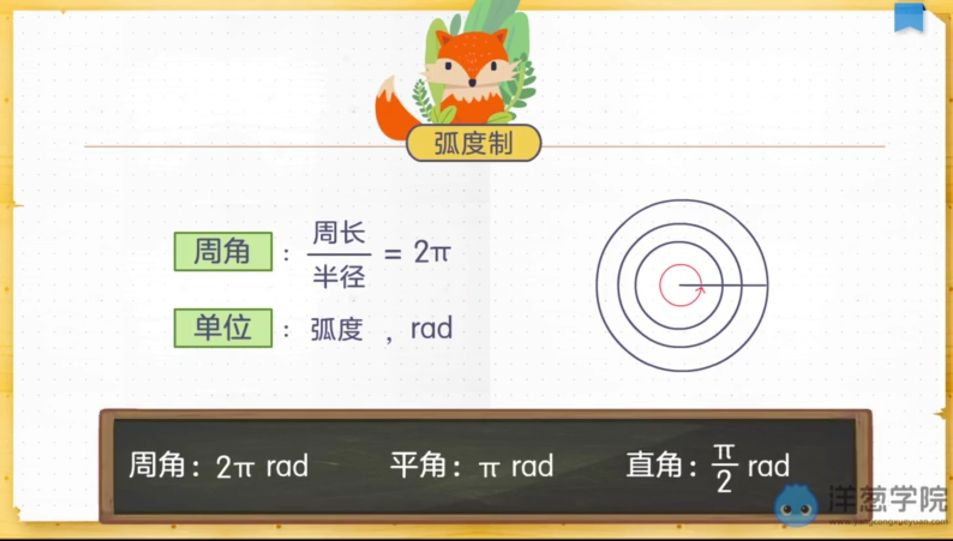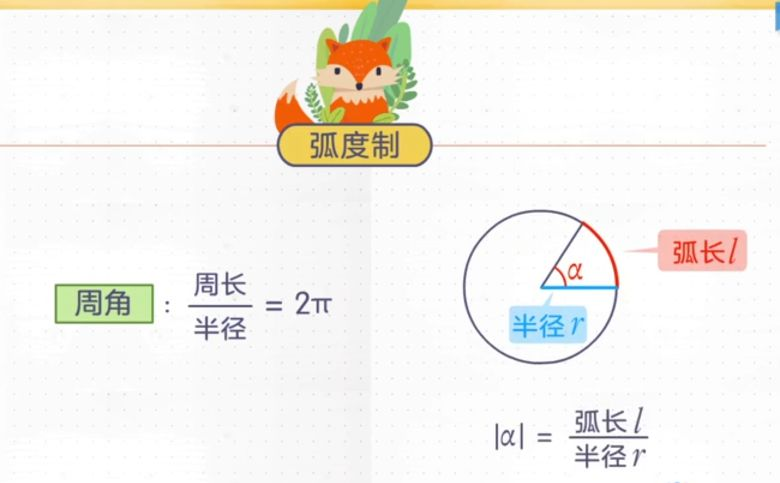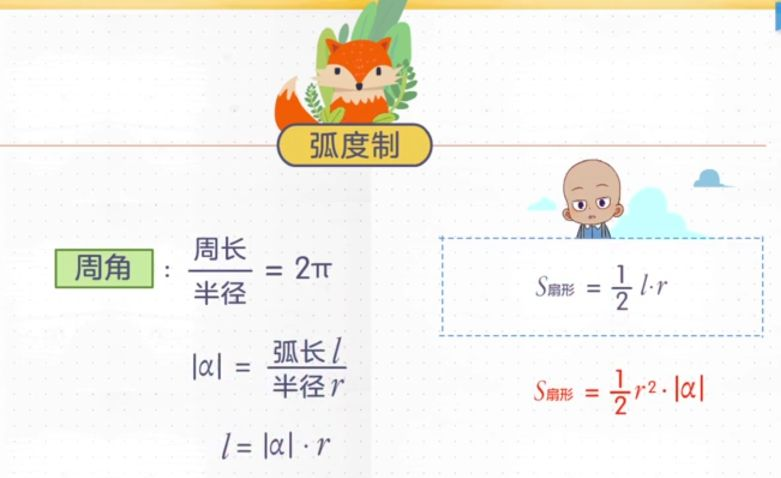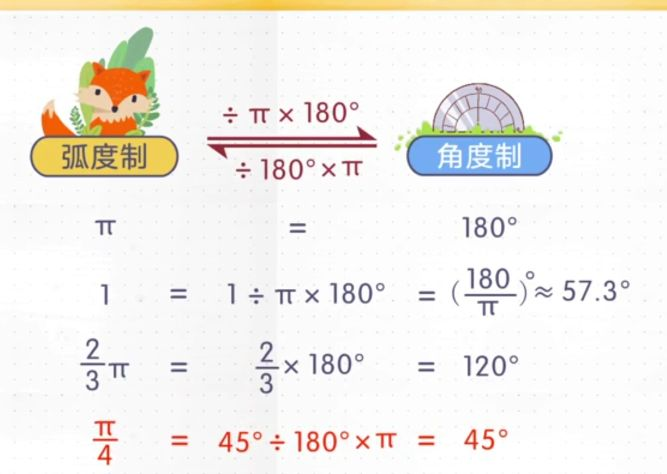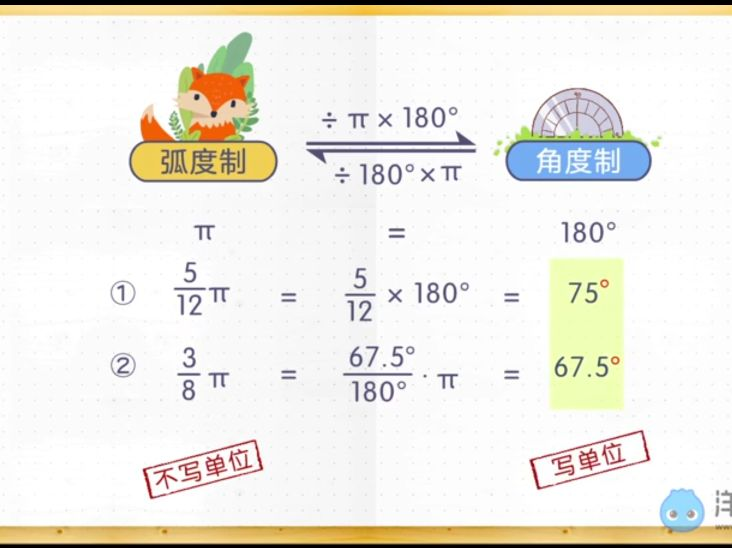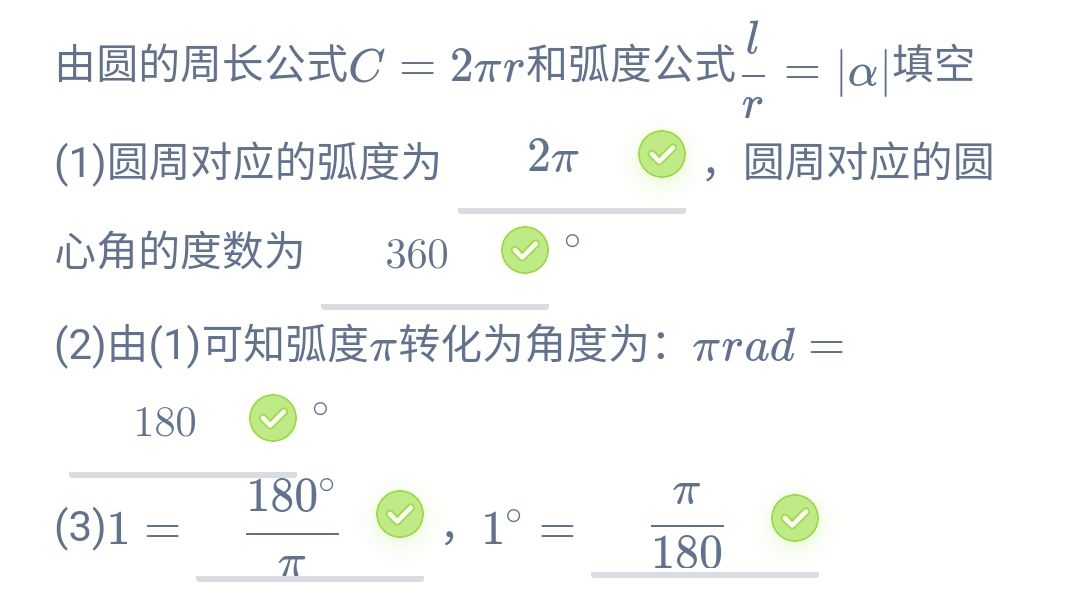二、任意角的三角函数
二(1)、三角函数的定义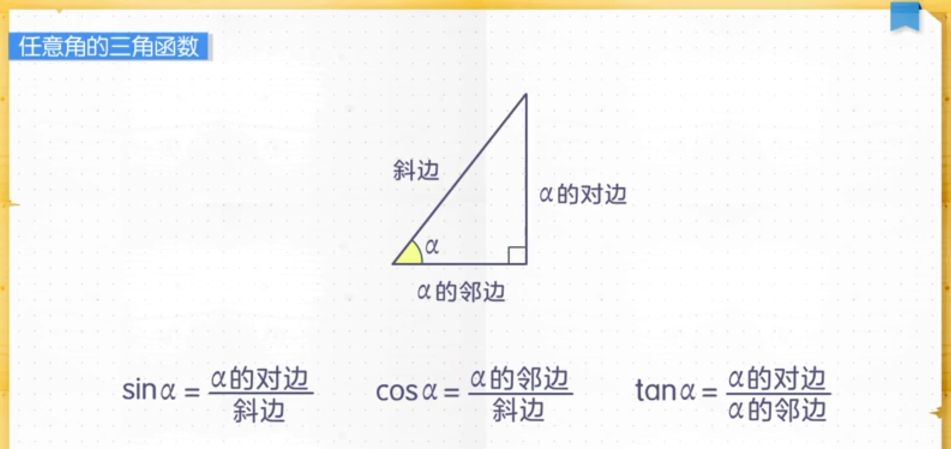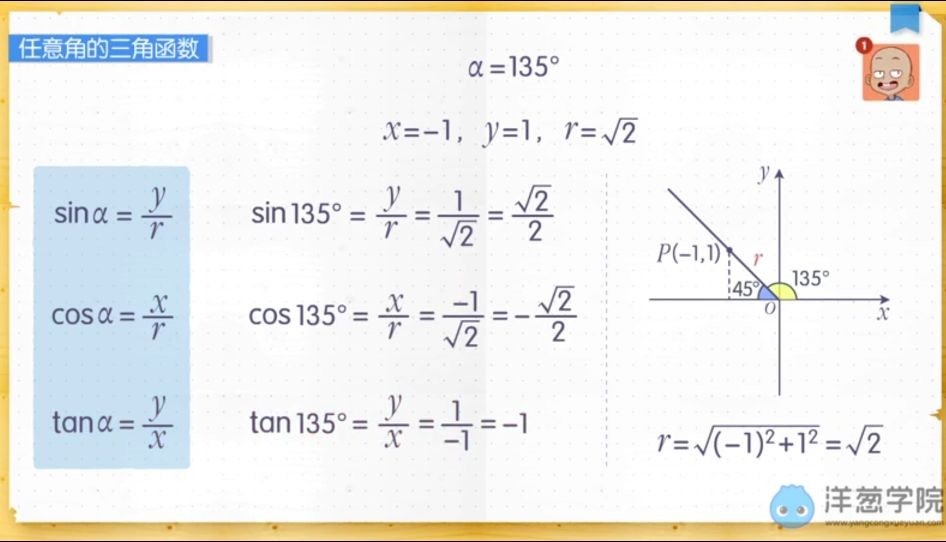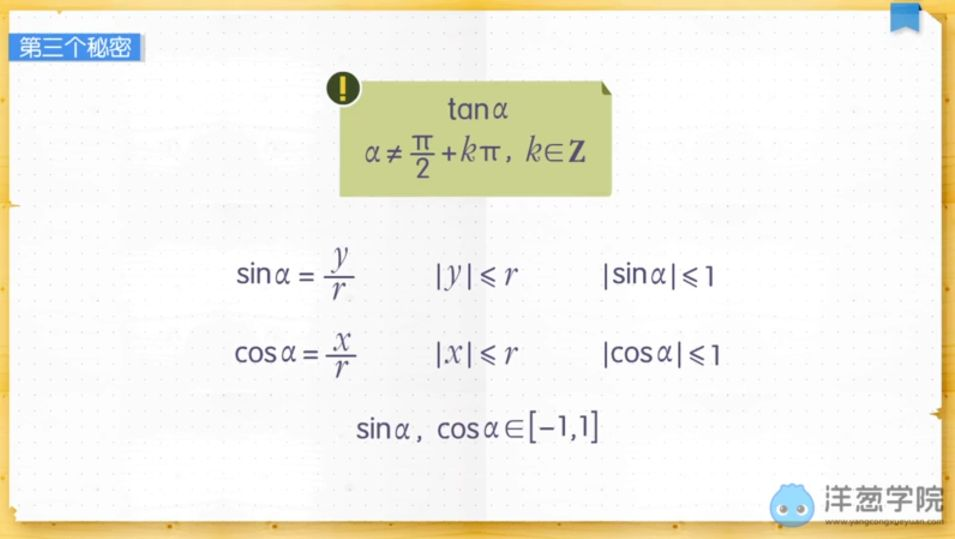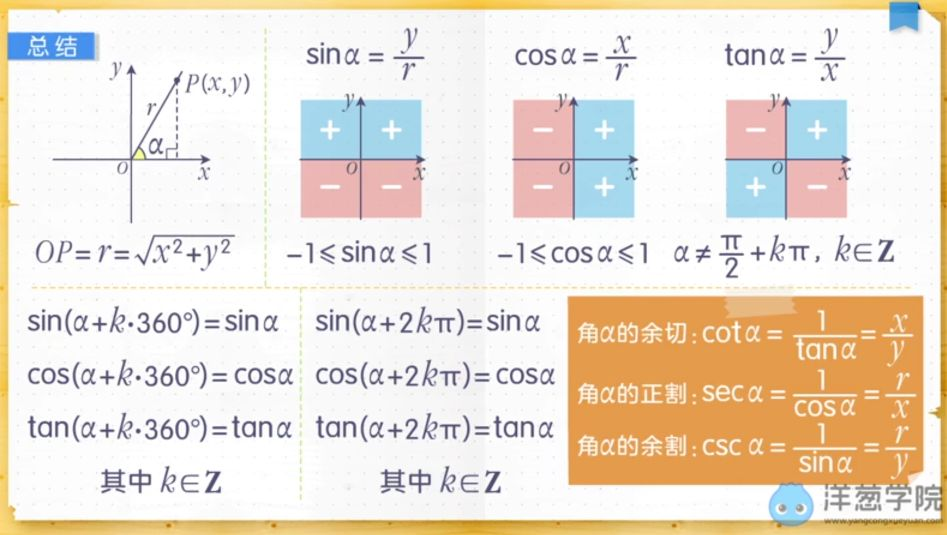二(2)、三角函数线
二(3)、同角三角函数的基本关系
二(4)、诱导公式
三、三角函数的图像与性质
二(1)正弦函数、余弦函数的图像与性质
二(1)1 正弦函数、余弦函数的图像
二(1)2 正弦函数、余弦函数的性质
二(2)、正余弦函数的图像与性质
二(2)1 y = sinx图像的三种变化
二(2)2 正余弦型函数的图象
二(2)3 画正余弦型函数的图象五点作图法
二(2)4 正余弦型函数的定义域、值域和周期
二(2)5 正余弦型函数的对称轴和对称中心
二(2)6 正余弦型函数的单调性
二(2)7 正余弦型函数的奇偶性
二(3)、正切函数的图像与性质
二(3)1 正切函数的图片与性质
文章目录
一、任意角的概念与弧度制一(1)、任意角一(2)、角度制与弧度制二、任意角的三角函数二(1)、三角函数的定义二(2)、三角函数线二(3)、同角三角函数的基本关系二(4)、诱导公式三、三角函数的图像与性质二(1)正弦函数、余弦函数的图像与性质二(2)、正余弦函数的图像与性质二(3)、正切函数的图像与性质


展开全文计算机视觉 opencv python 深度学习 算法
• 三角函数三角函数三角函数三角函数三角函数三角函数
• 三角函数的前面加上arc，表示它们的反函数f–1(x)。即由一个三角函数值得出当时的角度。 1. 正弦函数 sin x， 反正弦函数 arcsin x y = sin x， x∈R， y∈[–1，1]，周期为2π，函数图像以 x = (π/2) + k...
转载自:http://math001.com/inverse_trigonometric_functions/

在三角函数的前面加上 arc ，表示它们的反函数 f–1 (x)。即由一个三角函数值得出当时的角度。

1.  正弦函数 sin x， 反正弦函数 arcsin x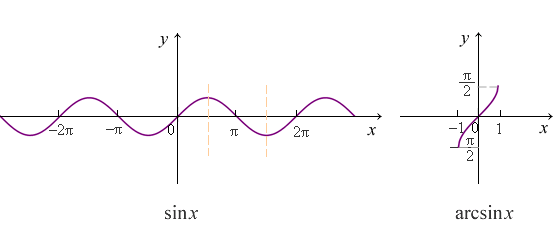y = sin x， x∈R， y∈[–1，1]，周期为2π，函数图像以 x = (π/2) + kπ 为对称轴
y = arcsin x， x∈[–1，1]， y∈[–π/2，π/2]
sin x = 0    ←→     arcsin x = 0
sin x = 1/2     ←→     arcsin x = π/6
sin x = √2/2    ←→     arcsin x = π/4
sin x = 1    ←→     arcsin x = π/2

2.  余弦函数 cos x， 反余弦函数 arccos x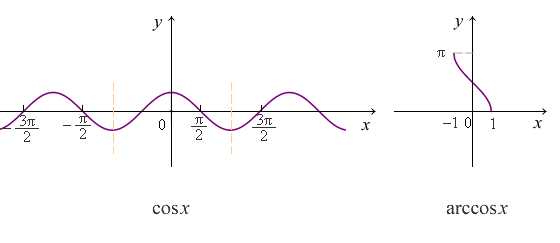y = cos x， x∈R， y∈[–1，1]，周期为2π，函数图像以 x = kπ 为对称轴
y = arccos x， x∈[–1，1]， y∈[0，π]
cos x = 0    ←→     arccos x = π/2
cos x = 1/2     ←→     arccos x = π/3
cos x = √2/2    ←→     arccos x = π/4
cos x = 1    ←→     arccos x = 0

3.  反正弦函数 arcsin x， 反余弦函数 arccos x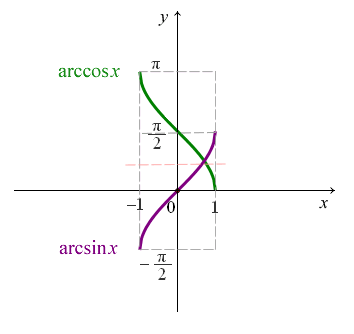y = arcsin x 与 y = arccos x 自变量的取值范围都是 x∈[–1，1]
y = arcsin x 与 y = arccos x 的图像关于直线 y = π/4 对称，相交与点 (√2/2 ，π/4)

4.   正切函数 tan x， 余切函数 cot x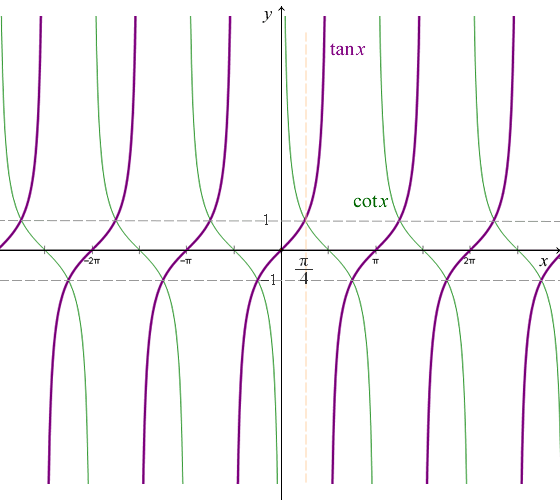y = tan x， x∈( (–π/2) + kπ， (π/2) + kπ )， y∈R，周期为π，当 x → ± (π/2) + kπ 时，函数的极限是无穷大 ∞
y = cot x = 1 / tan x， x∈( 0，kπ )， y∈R，周期为π，当 x →  kπ 时，函数的极限是无穷大 ∞
y = tan x 与 y = cot x 的图像关于 x =  (π/4) + kπ/2 对称
在单个周期内（第一个），y = tan x 与 y = cot x 的图像相交与点 （π/4 ，1）。当 x =  (π/4) + kπ/2 时，y = tan x 与 y = cot x 函数的值都相等，等于 ±1

5.   反正切函数 arctan x， 反余切函数 arccot x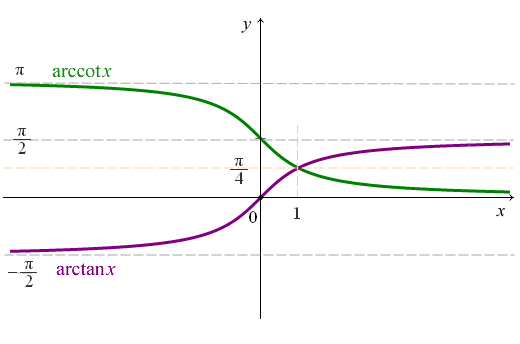y = arctan x 与 y = arccot x 自变量的取值范围都是 x∈R
y = arctan x 与 y = arccot x 的图像关于直线 y = π/4 对称，相交与点 (1 ，π/4)
tan x = 0    ←→     arctan x = 0
tan x = 1    ←→     arctan x = π/4
tan x = √3    ←→     arctan x = π/3

6.  余割函数 csc x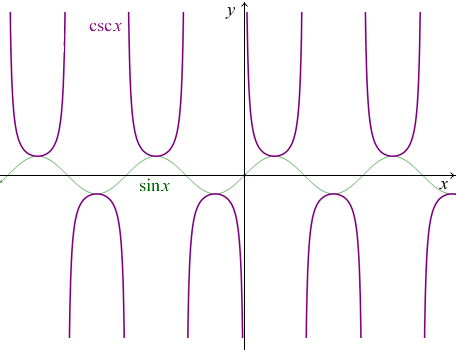y = csc x = 1 / sin x，x∈(0，kπ )， y∈(–∞，–1]∪[1，∞)，周期为π，当 x → kπ 时，函数的极限是无穷大 ∞

7.  正割函数 sec x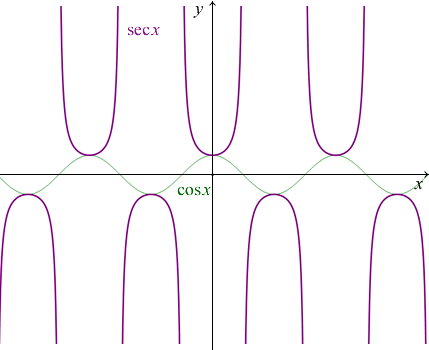y = sec x = 1 / cos x，x∈( (–π/2) + kπ， (π/2) + kπ )， y∈(–∞，–1]∪[1，∞)，周期为π，当 x → (π/2) + kπ 时，函数的极限是无穷大 ∞

展开全文考研数学
• 弧度制[课标解读][素养目标]借助单位圆理解三角函数(正弦、余弦、正切)的定义．水平一：1.借助单位圆理解并掌握任意角的三角函数(正弦、余弦、正切)定义(数学抽象).2.已知角α终边上一点，会求角α的各三角函数值...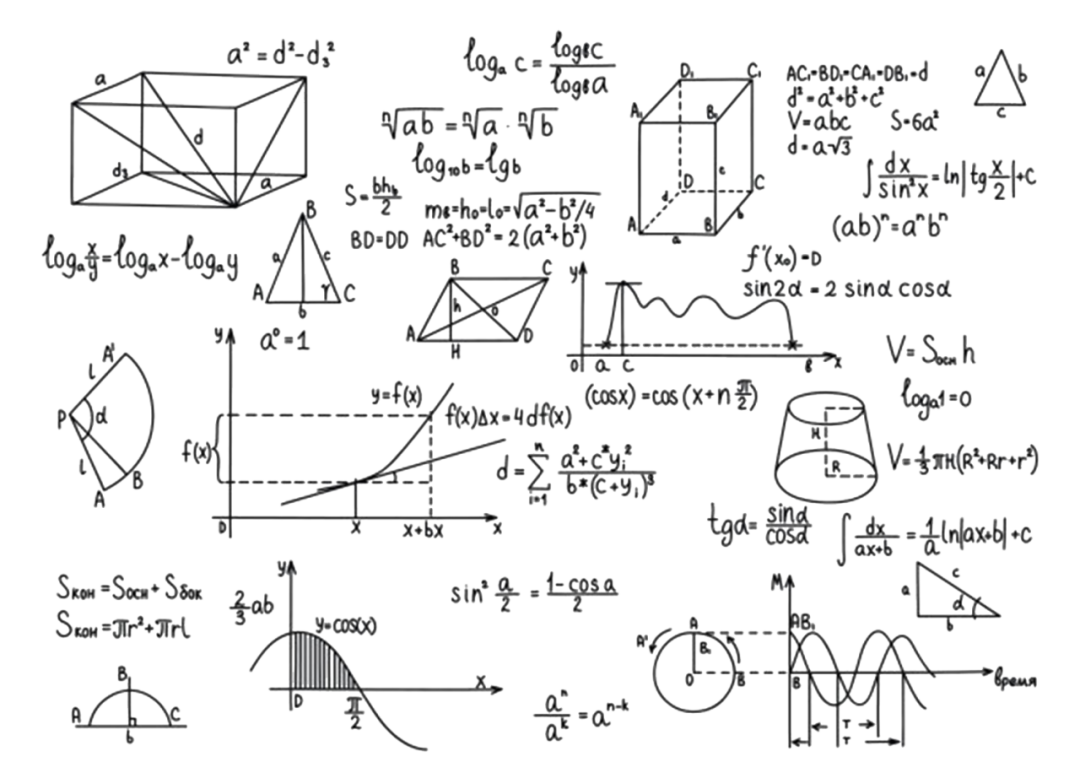弧度制[课标解读][素养目标]借助单位圆理解三角函数(正弦、余弦、正切)的定义．水平一：1.借助单位圆理解并掌握任意角的三角函数(正弦、余弦、正切)定义(数学抽象).2.已知角α终边上一点，会求角α的各三角函数值(数学运算)．水平二：使学生认识到事物之间是有联系的，三角函数就是角度(自变量)与比值(函数值)的一种联系方式(逻辑推理)．思考Think初中学习的锐角三角函数的定义是什么？点击下方空白区域查看答案▼「提醒」 如图，在Rt△ABC中，∠A，∠B，∠C的对边分别为a，b，c，则：sinB＝＝对边斜边，cosB＝＝邻边斜边，tanB＝＝对边邻边.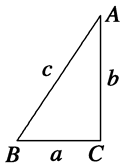思考Think对于确定的角α，请问三角函数的结果会随点P在α终边上的位置的改变而改变吗？点击下方空白区域查看答案▼「提醒」 根据相似三角形的知识，只要点P不与原点重合，三角函数值不会随P点在终边上的位置的改变而改变．本课须掌握的三大问题1．三角函数的定义(1)要明确sinα是一个整体，不是sin与α的乘积，它是“正弦函数”的一个记号，就如f(x)表示自变量为x的函数一样，离开自变量的“sin”“cos”“tan”是没有意义的．(2)任意一点P(x，y)：三角函数值是比值，是一个实数，由相似三角形的性质可知，这个实数的大小与点P的位置无关，只由角α的终边位置决定，即三角函数的大小只与角的终边位置有关．(3)由于角的集合与实数集之间可以建立一一对应关系，所以三角函数可以看成是自变量为实数的函数．2．定义法求三角函数值求角的三角函数值需要确定三个量：角的终边上异于原点的点的横、纵坐标及该点到原点的距离．一般地，在默认始边与x轴的非负半轴重合，顶点为原点的条件下，若已知角α的终边上的点或终边所在的位置，可直接利用定义求三角函数值．寄语Send word每个人的一天都是24小时，使用方式却千差万别。错把忙碌当高效，往往事倍功半；懂得高效做事，生活便能容得下更多美好与充实。时间会掌控，生活能自律，做事有效率，迎接你的将是最为美好的蜕变。新的一天，一起加油吧展开全文...